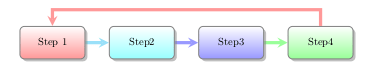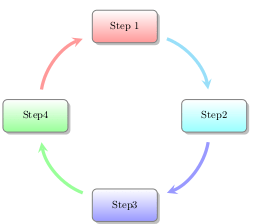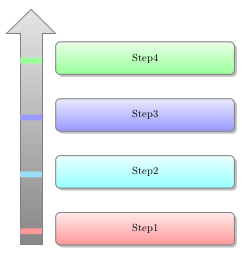# Drawing Diagrams in LaTeX

There is an old saying that most of us are familiar with that says that “a picture is worth a thousand words.” Knowing means that a communicating cannotonly include text but most also incorporate visuals as well. LaTeX allows you to develop visuals and diagrams using various packages for this purpose.

The visuals we will make are similar to those found in Microsoft Word Smart Graphics. One of the main advantages of using code to make diagrams is that they are within the document and you do not need to import images every single time you compile the document. If the image disappears it will not work but as long as the code is where you can always regenerate it.

In this post, we will use the “smartdiagram” package to make several different visuals that can be used in LaTeX. The types we will make are as follows…

• Flow diagram
• Circular diagram
• Bubble diagram
• Constellation diagram
• Priority diagram
• Descriptive diagram

The code for each individual diagram is almost the same as you will see. The preamble will only include the document class of “article” as well as the package “smartdiagram”. After this, we will create are document environment. Below is the preamble and the empty document environment.

\documentclass{article}
\usepackage{smartdiagram}
\begin{document}
\end{document}

Flow Diagram

The flow diagram is a diagram using boxes with arrows pointing from left to right in-between each box until the last box has an arrow that points back to the first bo indicating a cyclical nature. Below is  the code followed by the diagram

\smartdiagram[flow diagram:horizontal]{Step 1,Step2,Step3,Step4}The syntax is simple.

1. Call the declaration “\smartdiagram”
2. Inside the brackets, you indicate the type of diagram which was “flow diagram: horizontal” for us.
3. Next, you indicate how many boxes by typing the text and separating them by commas inside the curly braces.

This pattern holds for most of the examples in this post.

Circular Diagram

Below is a circular diagram. The syntax for the code is the same. Therefore, the code is below followed by the diagram

\smartdiagram[circular diagram:clockwise]{Step 1,Step2,Step3,Step4}Bubble Diagram

The same syntax as before. Below is the code and diagram.

\smartdiagram[bubble diagram]{Step 1,Step2,Step3,Step4}Constellation Diagram

This diagram looks similar to the bubble diagram but has arrows jutting out of the center. The syntax is mostly the same.

\smartdiagram[constellation diagram]{Step 1,Step2,Step3,Step4}Priority Descriptive Diagram

This diagram is useful if the order matters

\smartdiagram[priority descriptive diagram]{Step1, Step2, Step3,Step4}Descriptive Diagram

The coding for the descriptive diagram is slightly different. Instead of one set of curly braces, you have a set of curly braces within a set of curly braces within a final set of curly braces. The outer layer wraps the entire thing. The second layer is for circles in the diagram and the inner curly braces are for adding text to the rectangle. Each double set of curly braces are separated by a comma. Below is the code followed by the diagram.

\smartdiagram[descriptive diagram]{
{Step 1,{Sample text, Sample text}},
{Step2,{More text, more text}},
{Step3,{Text again, text again}},
{Step4,{Even more text}}
}Hopefully, you can see the formatting of the code and see how everything lines up.

Conclusion

Developing diagrams for instructional purposes is common in many forms of writing. Here, we simply look at creating diagrams using LaTeX. The power of this software is the ability to create almost whatever you need for communication.